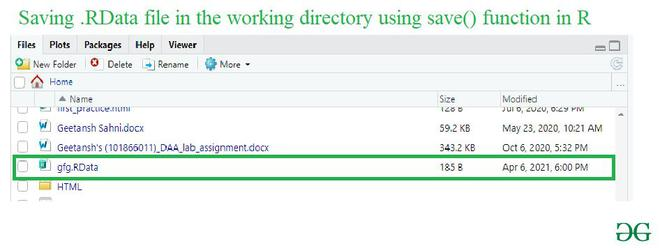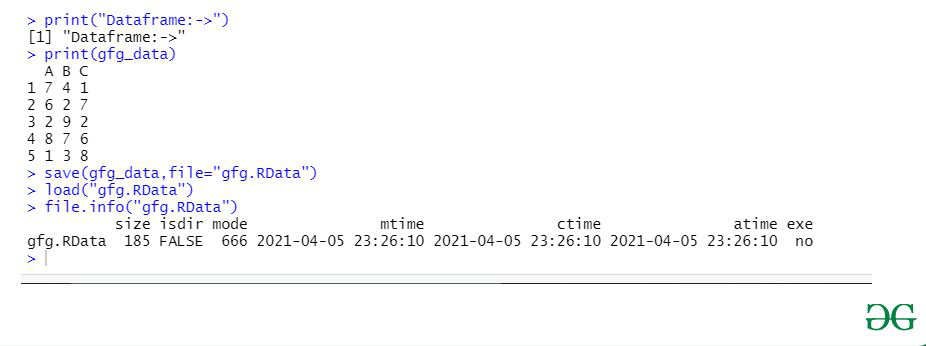How to export dataframe to RDATA file in R ?

• Last Updated : 17 Apr, 2021

In this, article we are going to save the information of a data frame in a RDATA file and display the information of the file using R Programming language. To save the information of a data frame in a file and display the information of the file in R language is as follows:

1. Using the save function to save the file in.RData format.
3. Using file.info() function to get the information of a particular file.

Step 1: Using the save() function to save the file in.RData format

In this step user need to call the save(), function with the name of the new file and with its format passed as its parameter, This function will simply save the file as per the user-specified parameter in the working directory.

save() function: This function writes an external representation of R objects to the specified file.

Syntax: save(…, list = character(), file = stop(“‘file’ must be specified”),ascii = FALSE, version = NULL, envir = parent.frame(), compress = isTRUE(!ascii), compression_level, eval.promises = TRUE, precheck = TRUE)

Example:

In this example, we will be simply saving a data frame in new.RData file in the working directory.

R

 gfg_data = data.frame(A = c(7,6,2,8,1),                     B = c(4,2,9,7,3),                     C = c(1,7,2,6,8))  print("Dataframe:->")   print(gfg_data)save(gfg_data, file = "gfg.RData")

Output:In this step user just need to call the load() function with the name of the file saved in step-1 as its parameter, further this will help to load the save file in the console so that the user can manage the operation on this file.

Load() function: This function is used to reload datasets written with the function save.

Syntax: load(file, envir = parent.frame(), verbose = FALSE)

Parameters:

• File:-a character string giving the name of the file to load.
• envir:-the environment where the data should be loaded.

Example:

Under this example, we will be loading the .RData file using load() function which was saved in the previous example.

R

 gfg_data= data.frame(A=c(7,6,2,8,1),                     B=c(4,2,9,7,3),                     C=c(1,7,2,6,8))  print("Dataframe:->")   print(gfg_data)  save(gfg_data,file="gfg.RData")load("gfg.RData")

Step3: Using file.info() function to get the information of a particular file.

This is the final step to save the information of a data frame in a file and displays its information, Here the step1 and step2 is used to save the information of the given data-frame in a particular file and this step3 will be used to displaying the information of the file where the given data-frame is been saved and for this, we will be using the file.info() function with the name of the previously loaded file in the console to display the information of the file saved.

file.info() function: This is a utility function used to extract information about files on the user’s file systems.

Syntax: file.info(…, extra_cols = TRUE)

Parameter:

• …:-character vectors containing file paths
• extra_cols:-Logical: return all cols rather than just the first six.

Returns: save

The complete information of the file including – size, mode, ctime, exe, time, atime, and isdir.

Example:

Using the file.info() function in R language we will be displaying the completed information of the file save and loaded in the previous examples.

R

 gfg_data= data.frame(A=c(7,6,2,8,1),                     B=c(4,2,9,7,3),                     C=c(1,7,2,6,8))  print("Dataframe:->")   print(gfg_data)  save(gfg_data,file="gfg.RData")load("gfg.RData")file.info("gfg.RData")

Output:My Personal Notes arrow_drop_up